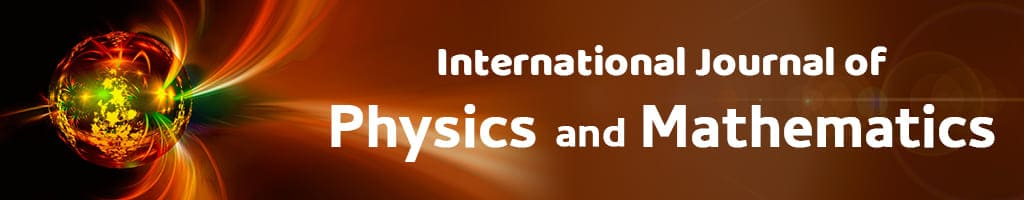# Vol. 2, Issue 1, Part A (2020)

## The Solutions of Selected Problems from IMO

Author(s):

Zhang Yue

Abstract:
Using the elementary theory of the inequality, the paper obtains all the real numbers x which satisfy the inequality of the problem 1; on the basis of the expanding formula of cosine of the angle 2x, it explicitly solves the equation of problem 2, and obtains all the solutions which satisfy the equation of the problem; it proves the equality of the problem 3 by use of the mathematical induction; moreover, it solves and obtains all the solutions (x1,x2,x3,x4) of problem 4; using the mathematical induction, the paper proves the conclusion of the problem 5; and finally, the paper offers a simple and rigorous proof on the problem 6.